# SCERT Maharashtra Question Bank solutions for 10th Standard SSC Science and Technology 2 Maharashtra State Board 2021 chapter 5 - Towards Green Energy [Latest edition]

#### Chapters## Chapter 5: Towards Green Energy

Choose the correct optionFind an odd one outName the followingRelate the followingWrite the function ofWrite true or falseMatch the followingDefineAnswer in one sentenceGive scientific reasonsWrite short notesDistinguish betweenAnswer the following questions
Choose the correct option

### SCERT Maharashtra Question Bank solutions for 10th Standard SSC Science and Technology 2 Maharashtra State Board 2021 Chapter 5 Towards Green Energy Choose the correct option

#### Choose the correct option.

Choose the correct option | Q 1

In modern civilization, __________ has become a primary need.

• food

• cloth

• shelter

• energy

Choose the correct option | Q 2

Most electric power plants are based on the principle of ___________.

• electro induction

• magnetic induction

• electromagnetic induction

• electromagnet

Choose the correct option | Q 3

Principle of Electromagnetic induction was invented by the scientist ___________.

• Ohm

• Joule

• Newton

Choose the correct option | Q 4

In the power plant based on nuclear energy, __________ is used to rotate the generator.

• Steam turbine

• air turbine

• water turbine

• none of these

Choose the correct option | Q 5

When a neutron is bombarded on an atom of uranium, ____________ neutrons generated in this process.

• 1

• 2

• 3

• 4

Choose the correct option | Q 6

Kinetic energy in flowing water drives ________ to generate electricity.

• watermill

• windmill

• turbines

• generator

Choose the correct option | Q 7

Wind turbines with capacity are commercially available.

• 1 kW to 7 MW

• 1 kW to 7 kW

• 1 kW to 7000 W

• 1 W to 7 MW

Choose the correct option | Q 8

Solar photovoltaic cells convert the solar radiation energy directly into _________ energy.

• electrical

• potential

• kinetic

• heat

Choose the correct option | Q 9

A silicon solar cell of dimension 1 sq.cm. generates a current of about ___________.

• 50 mA

• 30 mA

• 50 A

• 30 A

Choose the correct option | Q 10

A silicon solar cell of dimension 1 sq.cm. generates __________ potential difference.

• 0.1 V

• 0.5 V

• 0.1 mV

• 0.5 mV

Choose the correct option | Q 11

In nuclear power plants, neutrons are bombarded on atoms of __________.

• Uranium - 236

• Barium

• Krypton

• Uranium - 235

Find an odd one out

### SCERT Maharashtra Question Bank solutions for 10th Standard SSC Science and Technology 2 Maharashtra State Board 2021 Chapter 5 Towards Green Energy Find an odd one out

Find an odd one out | Q 1

Find an odd one out.

• Hydroelectric energy

• Solar energy

• Nuclear energy

• Wind Energy

Find an odd one out | Q 2

Find an odd one out.

• Silicon

• Uranium

• Petrol

• Coal

Find an odd one out | Q 3

Find an odd one out.

• Solar Photovoltaic cell

• Electricity generation using wind energy

• Hydroelectric power plant

• Solar thermal power plant

Find an odd one out | Q 4

Find an odd one out.

• Water

• Wind

• Natural gas

• Fossil fuel

Find an odd one out | Q 5

Find an odd one out.

• Edible oil

• LPG

• CNG

• Crude oil

Find an odd one out | Q 6

Find an odd one out.

• Coal

• Natural gas

• Plutonium

• Crude oil

Name the following

### SCERT Maharashtra Question Bank solutions for 10th Standard SSC Science and Technology 2 Maharashtra State Board 2021 Chapter 5 Towards Green Energy Name the following

#### Name the following.

Name the following | Q 1

The Electric Power Stations based on Thermal Energy.

Name the following | Q 2

Nuclear power plants in India

Name the following | Q 3

Major hydroelectric power plants in India

Name the following | Q 4

Forms of energy.

Name the following | Q 5

A machine/An engine required to rotate the generator.

Name the following | Q 6

Name any two natural gases.

Name the following | Q 7

Type of power generation station at Chandrapur.

Relate the following

### SCERT Maharashtra Question Bank solutions for 10th Standard SSC Science and Technology 2 Maharashtra State Board 2021 Chapter 5 Towards Green Energy Relate the following

#### Relate the following.

Relate the following | Q 1

Hydroelectric power station : Water stored in dams : : Nuclear power station : ____________

Relate the following | Q 2

Stove : Thermal energy : : Sewing machine : _____________

Relate the following | Q 3

Power available from solar cells : DC : : power require to run domestic equipments : _________

Relate the following | Q 4

Thermal power generation : Air pollution : : Nuclear power generation : _____________

Write the function of

### SCERT Maharashtra Question Bank solutions for 10th Standard SSC Science and Technology 2 Maharashtra State Board 2021 Chapter 5 Towards Green Energy Write the function of

Write the function of | Q 1

Write the function of Generator.

Write the function of | Q 2

Write the function of Turbine.

Write the function of | Q 3

Write the function of Condenser.

Write true or false

### SCERT Maharashtra Question Bank solutions for 10th Standard SSC Science and Technology 2 Maharashtra State Board 2021 Chapter 5 Towards Green Energy Write true or false

#### Write whether true or false.

Write true or false | Q 1

The power produced from solar cells is a DC.

• True

• False

Write true or false | Q 2

Electrical energy can be generated using wind.

• True

• False

Write true or false | Q 3

While producing hydroelectric energy, there is no pollution.

• True

• False

Write true or false | Q 4

In a windmill, the rotating blades drive the turbine and the turbine in turn drives the generator to generate electricity.

• True

• False

Write true or false | Q 5

In nuclear power plants, the chain reaction cannot be controlled.

• True

• False

Write true or false | Q 6

The disposal of nuclear waste safely is a big challenge before scientists.

• True

• False

Write true or false | Q 7

Efficiency of power generation plants based on energy of natural gas is higher than that of the power generation plant based on coal.

• True

• False

Write true or false | Q 8

In a hydroelectric power plant, the potential energy in water stored in a dam is converted into kinetic energy.

• True

• False

Write true or false | Q 9

In nuclear power plants, turbines working on solar panels are used.

• True

• False

Write true or false | Q 10

The potential difference available from a solar cell depends on its area.

• True

• False

Match the following

### SCERT Maharashtra Question Bank solutions for 10th Standard SSC Science and Technology 2 Maharashtra State Board 2021 Chapter 5 Towards Green Energy Match the following

Match the following | Q 1

Match the following.

 Column A Column B 1) Polluting energy a) smoke particles 2) Environment friendly energy b) thermal energy c) wind energy
Match the following | Q 2

Match the following.

 Column A Column B 1) Sunlight a) Wind energy 2) Natural gas b) Environment friendly energy c) Fossil fuel d) Atomic radiation
Define

### SCERT Maharashtra Question Bank solutions for 10th Standard SSC Science and Technology 2 Maharashtra State Board 2021 Chapter 5 Towards Green Energy Define

Define | Q 1

Define.

Electromagnetic induction

Define | Q 2

Define.

Nuclear fission

### SCERT Maharashtra Question Bank solutions for 10th Standard SSC Science and Technology 2 Maharashtra State Board 2021 Chapter 5 Towards Green Energy Answer in one sentence

Answer in one sentence | Q 1

Write the advantages of the Hydroelectric Power project.

Answer in one sentence | Q 2

Write the problems associated with hydroelectric power projects.

Answer in one sentence | Q 3

Write the merits of nuclear energy.

Answer in one sentence | Q 4

Write the demerits of a nuclear energy plant.

Answer in one sentence | Q 5

Draw the flowchart showing generation of electrical energy through hydroelectric power plant.

Answer in one sentence | Q 6

Which substance is used as fuel in nuclear power plants?

Answer in one sentence | Q 7

Energy from fossil fuels and nuclear plants are not eco-friendly sources, why?

Answer in one sentence | Q 8

Solar reflectors are important, why?

Answer in one sentence | Q 9

In fuels coal and natural gas, which one is eco-friendly? why?

Answer in one sentence | Q 10

State the importance of inverters.

Answer in one sentence | Q 11

Why are control rods used in nuclear reactors?

Answer in one sentence | Q 12.1

What is green energy?

Answer in one sentence | Q 12.2

Which energy source can be called a green energy source?

Answer in one sentence | Q 13

Explain: Fossil energy is not an example of green energy.

Give scientific reasons

### SCERT Maharashtra Question Bank solutions for 10th Standard SSC Science and Technology 2 Maharashtra State Board 2021 Chapter 5 Towards Green Energy Give scientific reasons

Give scientific reasons | Q 1

Give scientific reason.

The schematic of a turbine also varies according to the type of power generation.

Give scientific reasons | Q 2

Give scientific reason

It is absolutely necessary to control the fission reaction in nuclear power plants.

Give scientific reasons | Q 3

Give scientific reason

It is possible to produce energy from mW to MW using solar photovoltaic cells.

Give scientific reasons | Q 4

Give scientific reason.

Hydropower, solar energy and wind energy are called renewable energy.

Give scientific reasons | Q 5

Give scientific reason.

Energy saving is a need of the hour.

Write short notes

### SCERT Maharashtra Question Bank solutions for 10th Standard SSC Science and Technology 2 Maharashtra State Board 2021 Chapter 5 Towards Green Energy Write short notes

Write short notes | Q 1

Write a short note.

Lake Tapping

Write short notes | Q 2

Write a short note.

Nuclear Power Plant

Write short notes | Q 3

Write a short note.

Problems due to nuclear power plant

Distinguish between

### SCERT Maharashtra Question Bank solutions for 10th Standard SSC Science and Technology 2 Maharashtra State Board 2021 Chapter 5 Towards Green Energy Distinguish between

Distinguish between | Q 1

Explain the difference between Conventional and Non-conventional sources of energy.

Distinguish between | Q 2

Distinguish between the following.

Thermal power plant and Solar thermal power plant

Distinguish between | Q 3

Distinguish between the following.

Electricity generation from solar cells and solar thermal power generation

### SCERT Maharashtra Question Bank solutions for 10th Standard SSC Science and Technology 2 Maharashtra State Board 2021 Chapter 5 Towards Green Energy Answer the following questions

Answer the following questions | Q 1

Observe the given diagram and answer the questions given below.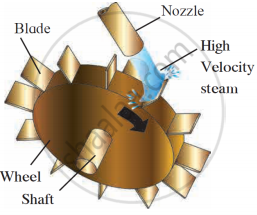1. What is the name of machine given in the diagram?
2. Write down the function of this machine in short.
3. How does it gets/forms the motion?
Answer the following questions | Q 2

Observe the given diagram and answer the questions.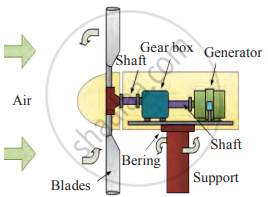1. Which type of energy is generated?
2. This power station depends on which factors?
3. Is it environment friendly?
Answer the following questions | Q 3

Complete the table.

Answer the following questions | Q 4.1

Complete the following flow chart.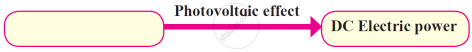Answer the following questions | Q 4.2

Complete the following flow chart.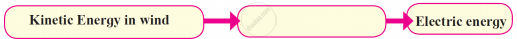Answer the following questions | Q 4.3

Complete the following flow chart.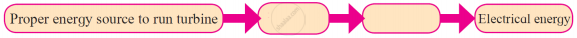Answer the following questions | Q 5.1

Draw the flowchart for the given power stations.

Thermal energy based electric power plant

Answer the following questions | Q 5.2

Draw the flowchart for the given power stations.

Power generation plant on energy of natural gas

Answer the following questions | Q 6

Explain the stages in electric generation from the given schematic diagram.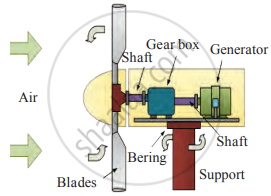Answer the following questions | Q 7

Draw the schematic diagram of natural gas power plants and answer the following questions.

1. Where is the power plant based on natural gas in Maharashtra?
2. Why is there less pollution by natural gas power plants?
3. Give the example of eco-friendly electric power generation plants.
Answer the following questions | Q 8

Solar reflectors are important, why?

Answer the following questions | Q 9

Complete the table.

 Limitations of Solar energy Limitations of Wind energy

## Chapter 5: Towards Green Energy

Choose the correct optionFind an odd one outName the followingRelate the followingWrite the function ofWrite true or falseMatch the followingDefineAnswer in one sentenceGive scientific reasonsWrite short notesDistinguish betweenAnswer the following questions## SCERT Maharashtra Question Bank solutions for 10th Standard SSC Science and Technology 2 Maharashtra State Board 2021 chapter 5 - Towards Green Energy

SCERT Maharashtra Question Bank solutions for 10th Standard SSC Science and Technology 2 Maharashtra State Board 2021 chapter 5 (Towards Green Energy) include all questions with solution and detail explanation. This will clear students doubts about any question and improve application skills while preparing for board exams. The detailed, step-by-step solutions will help you understand the concepts better and clear your confusions, if any. Shaalaa.com has the Maharashtra State Board 10th Standard SSC Science and Technology 2 Maharashtra State Board 2021 solutions in a manner that help students grasp basic concepts better and faster.

Further, we at Shaalaa.com provide such solutions so that students can prepare for written exams. SCERT Maharashtra Question Bank textbook solutions can be a core help for self-study and acts as a perfect self-help guidance for students.

Concepts covered in 10th Standard SSC Science and Technology 2 Maharashtra State Board 2021 chapter 5 Towards Green Energy are Energy and Use of Energy, Generation of Electrical Energy, Heat Energy (Thermal Energy), Nuclear Energy, Natural Gas Energy, Electric Energy Generation and Environment, Solar Energy, Solar Energy, Wind Energy, Hydroelectric Energy.

Using SCERT Maharashtra Question Bank 10th Standard [इयत्ता १० वी] solutions Towards Green Energy exercise by students are an easy way to prepare for the exams, as they involve solutions arranged chapter-wise also page wise. The questions involved in SCERT Maharashtra Question Bank Solutions are important questions that can be asked in the final exam. Maximum students of Maharashtra State Board 10th Standard [इयत्ता १० वी] prefer SCERT Maharashtra Question Bank Textbook Solutions to score more in exam.

Get the free view of chapter 5 Towards Green Energy 10th Standard [इयत्ता १० वी] extra questions for 10th Standard SSC Science and Technology 2 Maharashtra State Board 2021 and can use Shaalaa.com to keep it handy for your exam preparation